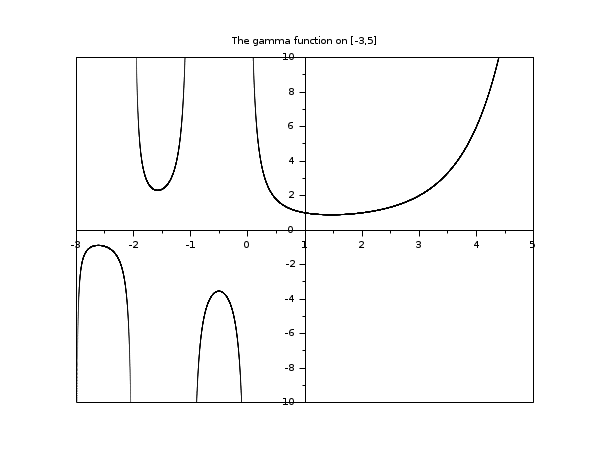Scilab Home page | Wiki | Bug tracker | Forge | Mailing list archives | ATOMS | File exchange
Scilab 5.5.2
Change language to: Français - Português - 日本語 - Русский

See the recommended documentation of this function

Scilab Help >> Special Functions > gamma

# gamma

The gamma function.

### Calling Sequence

`y = gamma(x)`

### Arguments

x

real vector or matrix

y

real vector or matrix with same size than x.

### Description

`gamma(x)` evaluates the gamma function at all the elements of `x`. The gamma function is defined by :and generalizes the factorial function for real numbers (`gamma(n+1) = n!`).

### Examples

```// simple examples
gamma(0.5)
gamma(6)-prod(1:5)```
```// the graph of the Gamma function on [a,b]
a = -3; b = 5;
x = linspace(a,b,40000)';
y = gamma(x);
clf()
c=xget("color")
xset("color",2)
plot2d(x, y, style=0, axesflag=5, rect=[a, -10, b, 10])
xset("color",c)
xtitle("The gamma function on ["+string(a)+","+string(b)+"]")
show_window()```• gammaln — The logarithm of gamma function.
• dlgamma — derivative of gammaln function, psi function

### History

 Version Description 5.4.0 Overloading allowed for list, mlist, tlist and hypermatrix types.